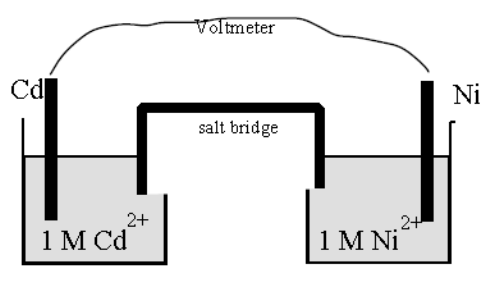# Problem: Ni-cad batteries are rechargeable and are commonly used in cordless appliances. Although such batteries actually function under basic conditions, imagine an electrochemical cell using the setup below. Cd2+(aq) + 2 e– → Cd (s)          Eº = – 0.40 VNi2+(aq) + 2 e– → Ni (s)            Eº = – 0.25 VWhat is E° for the cell?

###### Problem Details

Ni-cad batteries are rechargeable and are commonly used in cordless appliances. Although such batteries actually function under basic conditions, imagine an electrochemical cell using the setup below.Cd2+(aq) + 2 e → Cd (s)          Eº = – 0.40 V

Ni2+(aq) + 2 e → Ni (s)            Eº = – 0.25 V

What is E° for the cell?

Frequently Asked Questions

What scientific concept do you need to know in order to solve this problem?

Our tutors have indicated that to solve this problem you will need to apply the Electrochemical Cells concept. You can view video lessons to learn Electrochemical Cells. Or if you need more Electrochemical Cells practice, you can also practice Electrochemical Cells practice problems.

What is the difficulty of this problem?

Our tutors rated the difficulty ofNi-cad batteries are rechargeable and are commonly used in c...as low difficulty.

How long does this problem take to solve?

Our expert Analytical Chemistry tutor, Jules took 5 minutes and 12 seconds to solve this problem. You can follow their steps in the video explanation above.